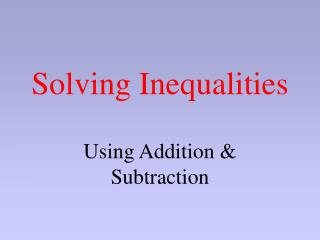Download PresentationSolving InequalitiesSolving Inequalities - PowerPoint PPT Presentation

Download PresentationSolving Inequalities
An Image/Link below is provided (as is) to download presentation

Download Policy: Content on the Website is provided to you AS IS for your information and personal use and may not be sold / licensed / shared on other websites without getting consent from its author. While downloading, if for some reason you are not able to download a presentation, the publisher may have deleted the file from their server.

- - - - - - - - - - - - - - - - - - - - - - - - - - - E N D - - - - - - - - - - - - - - - - - - - - - - - - - - -
Presentation Transcript

1. Solving Inequalities Using Addition & Subtraction

2. An inequality is like an equation, but instead of an equal sign (=) it has one of these signs: < : less than ≤ : less than or equal to > : greater than ≥ : greater than or equal to

3. “x < 5” means that whatever value x has, it must be less than 5. Try to name ten numbers that are less than 5!

4. -25 -20 -15 -10 -5 0 5 10 15 20 25 Numbers less than 5 are to the left of 5 on the number line. • If you said 4, 3, 2, 1, 0, -1, -2, -3, etc., you are right. • There are also numbers in between the integers, like 2.5, 1/2, -7.9, etc. • The number 5 would not be a correct answer, though, because 5 is not less than 5.

5. “x ≥ -2” means that whatever value x has, it must be greater than or equal to -2. Try to name ten numbers that are greater than or equal to -2!

6. -2 -25 -20 -15 -10 -5 0 5 10 15 20 25 Numbers greater than -2 are to the right of 5 on the number line. • If you said -1, 0, 1, 2, 3, 4, 5, etc., you are right. • There are also numbers in between the integers, like -1/2, 0.2, 3.1, 5.5, etc. • The number -2 would also be a correct answer, because of the phrase, “or equal to”.

7. -25 -20 -15 -10 -5 0 5 10 15 20 25 Where is -1.5 on the number line? Is it greater or less than -2? -2 • -1.5 is between -1 and -2. • -1 is to the right of -2. • So -1.5 is also to the right of -2.

8. Solve an Inequality w + 5 < 8 We will use the same steps that we did with equations, if a number is added to the variable, we add the opposite sign to both sides: w + 5 + (-5) < 8 + (-5) w + 0 < 3 All numbers less than 3 are solutions to this problem! w < 3

9. More Examples 8 + r ≥ -2 8 + r + (-8) ≥ -2 + (-8) r + 0 ≥ -10 w ≥ -10 All numbers from -10 and up (including -10) make this problem true!

10. More Examples x - 2 > -2 x + (-2) + (2) > -2 + (2) x + 0 > 0 x > 0 All numbers greater than 0 make this problem true!

11. More Examples 4 + y ≤ 1 4 + y + (-4) ≤ 1 + (-4) y + 0 ≤ -3 y ≤ -3 All numbers from -3 down (including -3) make this problem true!